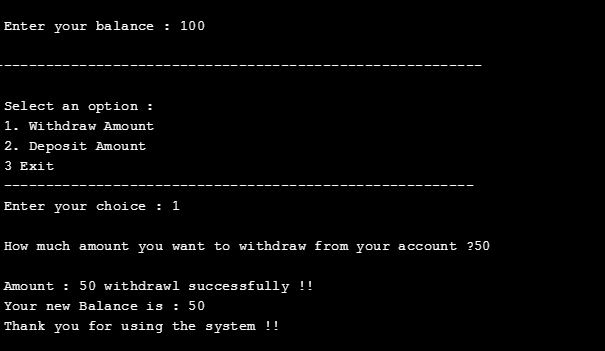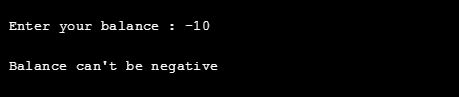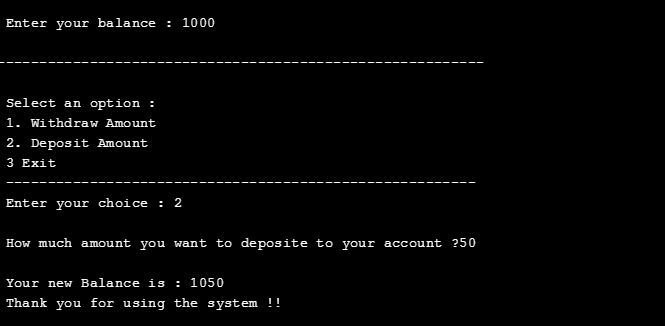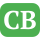CppBuzz.com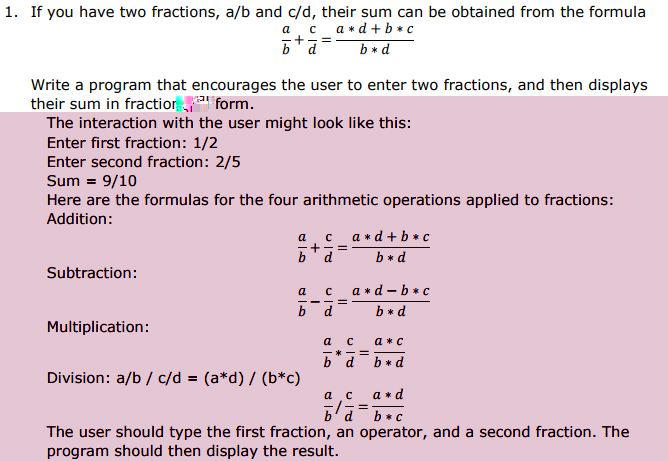Solution:- #include<iostream> #include<string.h> using namespace std; int main() { char firstFraction; //store max 4digits/4digits char secondFraction; //store max 4digits/4digits float a,b,c,d; cout<<"\n Enter first fraction: "; cin>>firstFraction; cout<<"\n Enter second fraction: "; cin>>secondFraction; if(strstr(firstFraction,"/")&&strstr(firstFraction,"/")) { char * ptr; ptr = strtok(firstFraction, "/"); a = atof(ptr); ptr = strtok(NULL, "/"); b = atof(ptr); ptr = strtok(secondFraction, "/"); c = atof(ptr); ptr = strtok(NULL, "/"); d = atof(ptr); cout<<"\n Sum ="<< (a*d +b *c) <<"/"<<(b*d); } else { cout<<"\nWrong Input.Format a/b"; } return 0; }
Output:-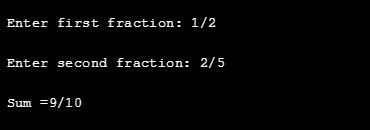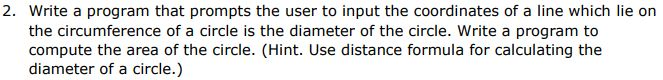Solution:- #include<iostream> #include<string.h> using namespace std; int main() { float x1,x2,y1,y2; float areaofCircle; float diameter; float radious; cout<<"\n Enter co ordiantes of a line :-"; cout<<"\n Enter x1 :"; cin>>x1; cout<<"\n Enter y1 :"; cin>>y1; cout<<"\n Enter x2 :"; cin>>x2; cout<<"\n Enter y2 :"; cin>>y2; //applying distance formual to calucale diameter diameter = sqrt((x2-x1)*(x2-x1) + (y2-y1)*(y2-y1)); radious = diameter/2; areaofCircle = 3.14 * radious * radious; cout<<"\n Area of a Circle is :" << areaofCircle; return 0; }
Output:-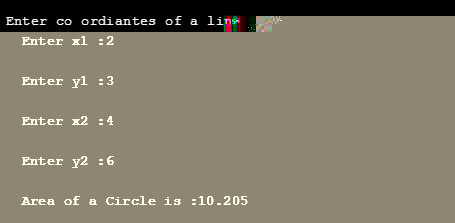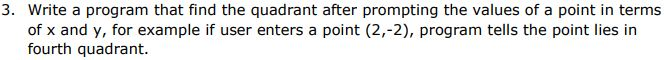Solution:-
#include<iostream> #include<string.h> using namespace std; int main() { float x,y; cout<<"\n Enter x : "; cin>>x; cout<<"\n Enter y : "; cin>>y; if( x < 0 && y < 0) { cout<<"\n Third Quadrant"; } else if( x > 0 && y > 0) { cout<<"\n First Quadrant"; } else if( x < 0 && y > 0) { cout<<"\n 2nd Quadrant"; } else if( x >0 && y < 0) { cout<<"\n 4th Quadrant"; } return 0; }
Output:-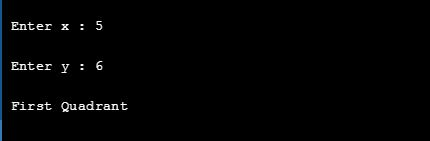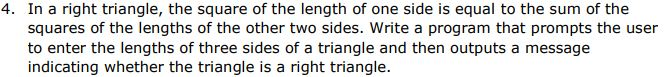Solution:- #include<iostream> #include<string.h> using namespace std; int main() { float Hypotenuse, Perpdendicular, Base; cout<<"\n Enter Hypotenuse :-"; cin>>Hypotenuse; cout<<"\n Enter Perpdendicular:- "; cin>>Perpdendicular; cout<<"\n Enter Base :-"; cin>>Base; if( (Hypotenuse*Hypotenuse) == ( (Perpdendicular*Perpdendicular) + (Base * Base)) ) { cout<<"\n Triangle is a Right angle triangle"; } else { cout<<"\n Triangle is not a Right angle triangle"; } return 0; }
Output:-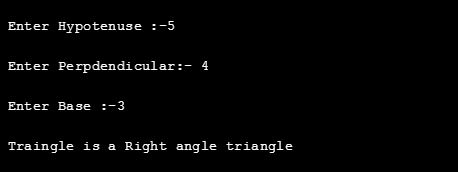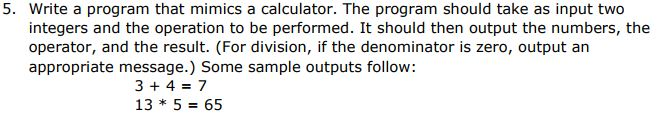Solution:- #include<iostream> #include<string.h> using namespace std; void Addition(float a, float b) { cout<<"\n Addition is ----> "<< a+b; } void Substraction(float a, float b) { cout<<"\n Substractionum is ----> "<< a - b; } void Multiplicatin(float a, float b) { cout<<"\n Multiplicatin is ----> "<< a * b; } void Division(float a, float b) { if( b== 0) cout<<"\n Divide by Zero error. B can't be 0"; else { cout<<"\n Division is ----> " << a/b; } } int main() { int choice = 1; while(choice != 0) { cout<<"\n\n ############# Calculator #############"; cout<<"\n\n Press 1 for Addition ( + ) "; cout<<"\n Press 2 for Substraction ( - ) "; cout<<"\n Press 3 for Multiplicatin ( * ) "; cout<<"\n Press 4 for Division( / ) "; cout<<"\n Press 0 exit"; cout<<"\n\n Enter your choice :"; cin>>choice; float a, b; cout<<"\n Enter a : "; cin>>a; cout<<"\n Enter b :"; cin>>b; switch(choice) { case 1: Addition(a, b); break; case 2: Substraction(a, b); break; case 3: Multiplicatin(a, b); break; case 4: Division(a, b);break; default: cout<<"\n Invalid Choice :("; } cout<<"\n Please enter to continue"; getchar(); } return 0; }
Output:-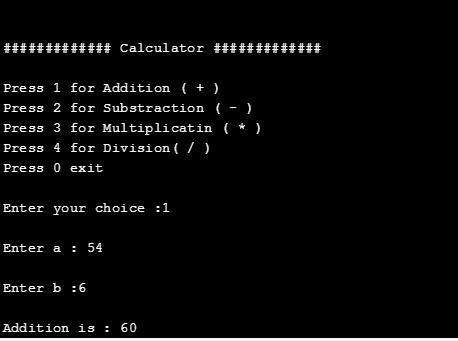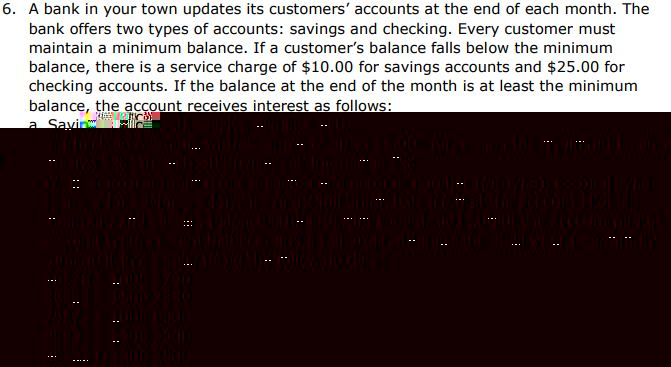Solution:- #include<iostream> using namespace std; int main() { int account_number; char account_type; double minimum_balance; double current_balance; cout<<"\n ###### Welcome to National Bank ######\n\n"; cout<<"\n Enter Account Number (Only Numberic Values) : "; cin>> account_number; cout<<"\n Enter Account Type ( s-> Saving, c -> checking): "; cin>> account_type; cout<<"\n Enter Minimum Balance : "; cin>>minimum_balance; cout<<"\n Enter Current Balance : "; cin>>current_balance; if( current_balance < minimum_balance) { cout<<"\n Note:=- Customer Account Balance less than Minimum Balance"; if( account_type== 's' || account_type== 'S') { cout<<"\n Customer has to pay \$10.00 As Customer holds Saving Account"; } else if( account_type== 'c' || account_type== 'C') { cout<<"\n Customer has to pay \$25.00 as Custome holds Checking Account"; } } else { cout<<"\n Note:=- Customer Maintains Minimum Balance in Account"; if( account_type== 's' || account_type== 'S') { cout<<"\n Customer applicable to get 4% ROI per year"; } else if( account_type== 'c' || account_type== 'C') { if(current_balance <= 5000) { cout<<"\n Customer applicable to get 3% ROI per year"; } else { cout<<"\n Customer applicable to get 5% ROI per year"; } } } return 0; } <xmp> <br>Output:-<br> <img src="images/qs/asg/output6.1.jpg" class="responsive" alt="C++ program to find ROI for customer"><br> <img src="images/qs/asg/output6.2.jpg" class="responsive" alt="C++ code to calcualte Rate of Interest"><br> <img src="images/qs/asg/qs7.jpg" class="responsive" alt="C++ Assignment on Electricity Bill Calculator"><br> <br> <br>Solution:- <xmp> #include<iostream> using namespace std; int main() { float units; float bill; cout<<"\n #### Electicity Bill Calculator #####\n"; cout<<"\n\n Enter your electicity bill units : "; cin>>units; if(units <= 100) { bill = units * 4; } else if( units >100 && units <=300) { bill = units * 4.5; } else if( units >300 && units <=500) { bill = units * 4.75; } else { bill = units * 5; } cout<<"\n Electicity bill : "<< bill; float fuel_surcharge = (bill *20)/100; float govt_tax = (bill *10)/100; cout<<"\n Fuel Surcharge : "<< fuel_surcharge; cout<<"\n Govt Tax : "<< govt_tax; cout<<"\n\n ## Total Electicity Bill : " << bill + fuel_surcharge + govt_tax; return 0; }
Output:-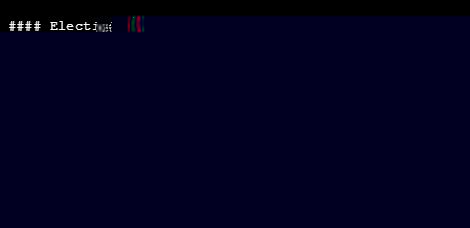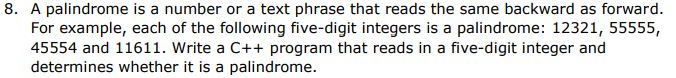Solution:- #include<iostream> using namespace std; int main() { long int n, num, digit, rev = 0; cout << "Enter a 5 digit positive number: "; cin >> num; n = num; do { digit = num % 10; rev = (rev * 10) + digit; num = num / 10; } while (num != 0); cout << " The reverse of the number is: " << rev << endl; if (n == rev) cout << " The number is a palindrome"; else cout << " The number is not a palindrome"; return 0; }
Output:-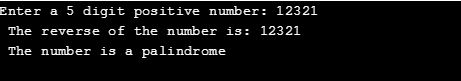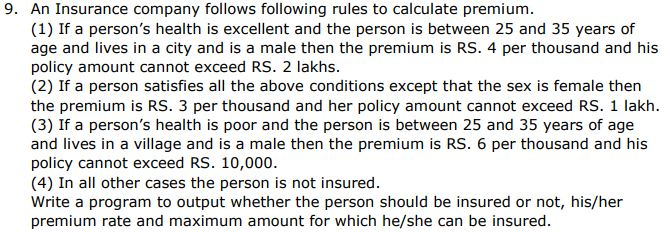Solution:- #include<iostream> #include<string.h> using namespace std; int main() { char health; // excellent, poor unsigned int age; // always postive number char locality; // either villege or city char sex; // M for Male, F for female unsigned premium; unsigned policy_amount; cout<<"\n ####### Check your Insurance Eligibility ######\n\n"; int value = 0; cout<<"\n Select Health "; cout<<"\n Press 1 for Excellent"; cout<<"\n Press 2 for Poor"; cout<<"\n\n Enter :"; cin>>value; if(value ==1) strcpy(health,"Excellent"); else if(value ==2) strcpy(health,"Poor"); cout<<"\n No Please enter age : "; cin>>age; cout<<"\n Please select locality "; cout<<"\n Press 1 for City "; cout<<"\n Press 2 for Villege"; cout<<"\n Enter ur locality : "; cin>>value; if(value ==1) strcpy(locality,"City"); else if(value ==2) strcpy(locality,"Villege"); cout<<"\n Enter your Sex (M/F) : "; cin>> sex; int flag = 0; if( strcmp(health, "Excellent") == 0) { if(sex =='M' || sex == 'm') { if(age>=25 && age <=35) { if(strcmp(locality, "City") == 0) { cout<<"\n Premium Rate is Rs. 4 Per Thousand"; cout<<"\n Max Policy Amonut is Rs. 2 Lacks"; flag = 1; } } } else if(sex =='F' || sex == 'f') { if(age>=25 && age <=35 && (strcmp(locality, "City") == 0)) { cout<<"\n Premium Rate is Rs. 3 Per Thousand"; cout<<"\n Max Policy Amonut is Rs. 1 Lack"; flag = 1; } } } else if(strcmp(health, "Poor") ==0) { if(sex =='M' || sex == 'm') { if(age>=25 && age <=35 && (strcmp(locality, "Villege") == 0)) { cout<<"\n Premium Rate is Rs. 6 Per Thousand"; cout<<"\n Max Policy Amonut is Rs. 10,000"; flag = 1; } } } if( flag ==0 ) { cout<<"\n You are not secured !!!"; } return 0; }
Output:-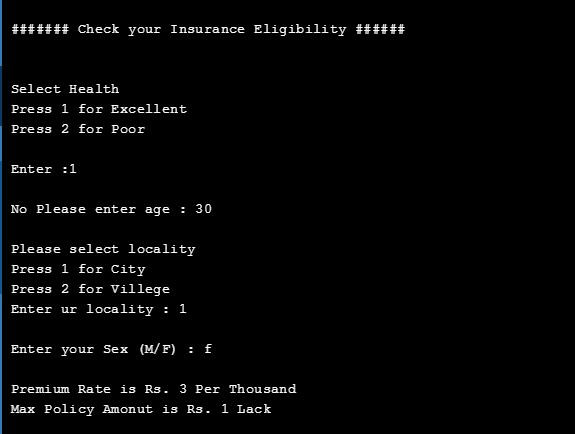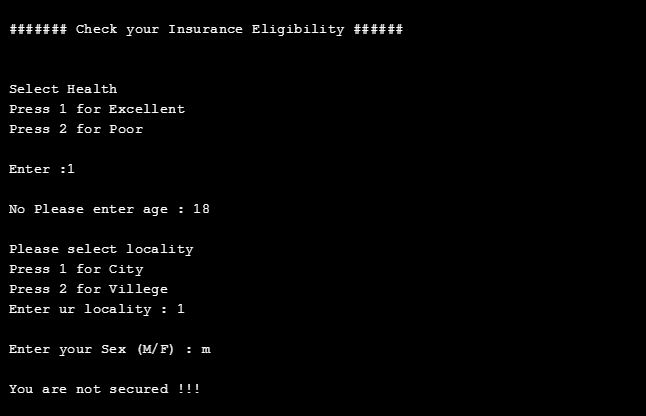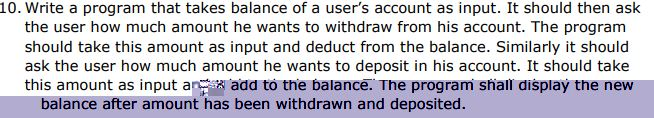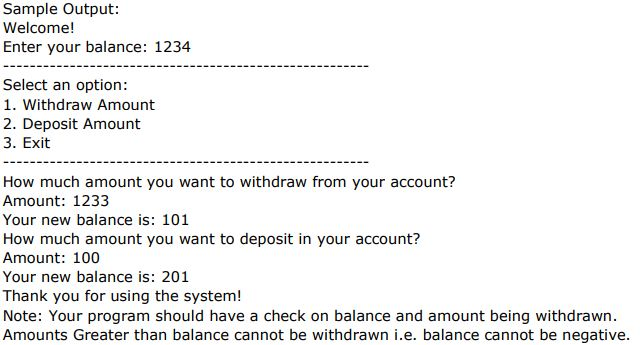Solution:- #include<iostream> using namespace std; int main() { float user_balance; float withdrawl_amount; float deposite_amount; cout<<"\n Enter your balance : "; cin>>user_balance; if(user_balance > 0) { cout<<"\n----------------------------------"; cout<<"\n\n Select an option : "; cout<<"\n 1. Withdraw Amount "; cout<<"\n 2. Deposit Amount "; cout<<"\n 3 Exit"; cout<<"\n ---------------------------------"; int choice = 0; cout<<"\n Enter your choice : "; cin>>choice; switch(choice) { case 1: cout<<"\n How much amount you want to withdraw from your account ? "; cin>>withdrawl_amount; if(withdrawl_amount > user_balance) cout<<"\n Fund Insufficient !!"; else { user_balance = user_balance - withdrawl_amount; cout<<"\n Amount : " <<withdrawl_amount <<" withdrawl successfully !!"; cout<<"\n Your new Balance is : "<< user_balance; } break; case 2: cout<<"\n How much amount you want to deposite to your account ? "; cin>>deposite_amount; user_balance = user_balance + deposite_amount; cout<<"\n Your new Balance is : "<< user_balance; break; default: cout<<"\n Invalid Choice !!"; } cout<<"\n Thank you for using the system !!"; } else { cout<<"\n Balance can't be negative "; } getchar(); return 0; }
Output:-